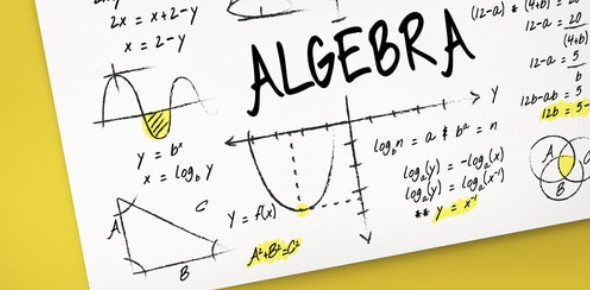# Algebra Questions: Practice Test! Quiz

12 Questions | Total Attempts: 278SettingsHow much do you know about algebra? Would you like answers to questions about algebra? Algebra is the study of mathematical symbols and rules pertaining to the use of these symbols. Algebra is notoriously difficult even with the best of teachers, but you can take this practice test to ensure that you are prepared for the real exam. If you need to ask algebra questions, this is the quiz that has the answers.

• 1.
The sum of twice r and three times s is identical to thirteen.
• A.

2r+13s=13

• B.

2(r+3s)=13

• 2.
Solve: 142+d=97
• 3.
Write an equation for the problem below: a number increased by 5 is equal to 42 (use x as your variable)
• 4.
Solve:18-(-f)=91
• 5.
Solve:b=-117
• 6.
-58=-29h
• 7.
Solve:-11=7+3c
• 8.
Solve:7g-14=63
• 9.
Solve:2m+5=5(m-7)-3m
• A.

M=1

• B.

M=0

• C.

M=All Reals

• D.

No Solution

• 10.
Solve:5t-9=-3t+7
• 11.
Solve: r   =2515  16
• 12.
A pizza store is having a sale on a large pizza.  The original price is 10 dollars.  The new price is 5 dollars.  What is the percent of change?
• A.

+50%

• B.

-50%

• C.

-10%

• D.

+10%

Related TopicsBack to top